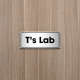# ABC Proof Could Be Mathematical Jackpot

You can’t always solve a mathematical problem by reducing it to something you’ve already solved. Sometimes, you need to invent an entirely new field of mathematics. Last month, Shinichi Mochizuki of Kyoto University in Japan announced that a new field he’s been developing for several years—which he calls Inter-universal Teichmüller theory—has proved a famous conjecture in number theory known as the “abc conjecture.” But the abc conjecture is only the beginning: If Mochizuki’s theory proves correct, it will settle a raft of open problems in number theory and other branches of math.

The conjecture grows out of a seemingly trivial equation: a + b = c. Unlike the equation a2 + b2 = c2, which requires some algebraic finesse to produce solutions (not to mention its famously unsolvable cousin a n + bn = cn with exponent n greater than two), the equation a + b = c essentially solves itself: Just pick two numbers a and b, add them together, and voilà.

But when you bring in prime numbers, things get interesting. In the mid 1980s, mathematicians David Masser of the University of Basel in Switzerland and Joseph Oesterlé of Pierre and Marie Curie University in Paris observed that when a and b are divisible by small primes raised to large powers—numbers such as a = 210 and b = 34—their sum c tends to factor into large primes to small powers. (In this example, 1024 + 81 = 1105 = 5 x 13 x 17.) The abc conjecture describes this connection in precise mathematical language, highlighting how the underlying “tension” between the operations of addition and multiplication produce such lopsided equations: many small primes on one side, a few relatively large primes on the other.

The excitement over Mochizuki’s potential proof is comparable to the frisson that greeted the announcement by Grigory Perelman in 2002 that he had proved the Poincaré conjecture, and by Andrew Wiles in 1993 that he had proved Fermat’s Last Theorem. But those proofs, although extremely complicated, were based on techniques that were broadly familiar to experts in the field. As a result, researchers could “helicopter in” on portions of the proofs to check their correctness.

Mochizuki, in contrast, has developed an entirely new theory—which will make it tougher for other mathematicians to scrutinize. “As with Perelman or Wiles, this is a first-class mathematician with a very strong reputation in the subject, who has been working on this important problem for many years, so the claim is certainly being taken very seriously,” writes mathematician Terence Tao of the University of California, Los Angeles, in an e-mail. “But it is likely to take quite some time to evaluate the work properly, more so than with either Perelman or Wiles.”

Mochizuki’s proof, Tao says, is built on decades of work in an extremely difficult area of mathematics known as anabelian geometry, which very few people in the world are actively working on. Launched in the 1980s, anabelian geometry seeks to ground mathematics in certain fundamental concepts—even more fundamental than the set theory mathematicians explored for that purpose in the 19th and 20th centuries.

Mochizuki’s Inter-universal Teichmüller theory is his own take on this reconceptualization. Given that his description of the theory—the last installment of which came out in August—spans four dense papers totaling about 500 pages, it will probably take other mathematicians months of study to understand the theory, let alone evaluate it or think about what other mathematical problems it might lay to rest.

“His work probes the very core of mathematical language, such as what we might really mean by a number or a geometric figure, and how they might be interpreted in a manner quite different from usual conventions,” writes Minhyong Kim of the University of Oxford in the United Kingdom in an e-mail. Kim, who is one of the world’s few experts in anabelian geometry, admits even he has his work cut out for him in mastering Mochizuki’s new techniques. “There is no one but the author who is familiar with all these things,” he writes. “I can’t even give an expert summary of the proof because I don’t understand it!”

—-

Barry Cipra

## One thought on “ABC Proof Could Be Mathematical Jackpot”

1.Sharing says: